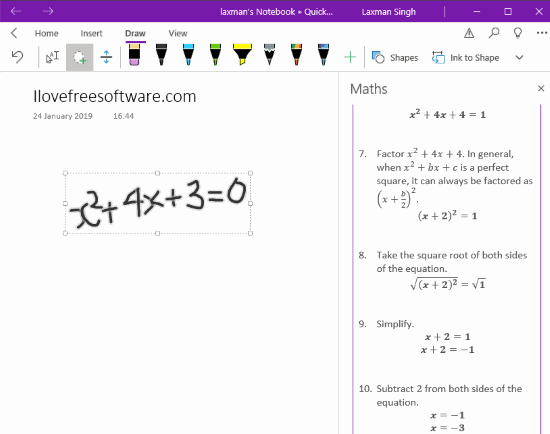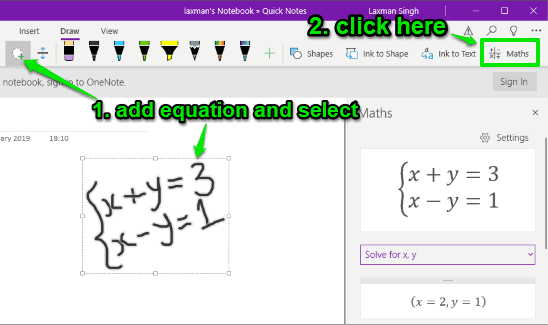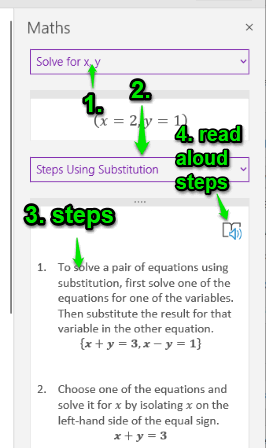Editor Ratings:
User Ratings:
[Total: 0 Average: 0]

This article covers how to solve Math equation with steps in OneNote and read aloud those steps. Both are the native features of OneNote, which have come recently in OneNote for Windows 10 and OneNote online. This article covers Windows 10 part.

Adding an equation using the pen is already a part of OneNote which comes under the Math section. And you are also able to get the solution and add it to the page. Now you can see the step by step instructions as well as read aloud those instructions (using Immersive Reader). You can also drag n drop instructions to the page so you will have multiple benefits.

In the screenshot added below, you can see that a Math equation is added and step by step instructions are available for that equation.## How to Solve Math Equation with Steps in OneNote, Read Aloud Steps?

Step 1: Open OneNote (writing about Windows 10 OneNote here).

Step 2: Access Draw menu and then use Pen to write an equation.

Step 3: When the equation is added, use “Lasso Select” tool and select the equation. After that, click on “Maths” option. See the screenshot below.Step 4: A sidebar will open on the right-hand side. There you will see the solution. Along with that, you will see Solve with drop-down menu (solve with x, solve with y, etc.). You can select an option and step by step instructions about how that equation is solved are visible to you.To read aloud the instructions, you can use “Immersive Reader” icon, as visible in the above screenshot. Though this feature is good, it didn’t work properly for me and I faced some error. You may try if it works.

You can also drag n drop the equations steps to the page to add the instructions there.

Did you know you can also add syntax highlighting to code in OneNote?

### The Conclusion:

This feature to solve Math equations in OneNote is pretty good. And now, you can see step by step instructions to solve the equation and add it to the page of your OneNote Notebook. Immersive Reader feature is also a nice addition to read aloud instructions but it needs some work to use without error.

 Editor Ratings: User Ratings:[Total: 0 Average: 0] Tags: math equation